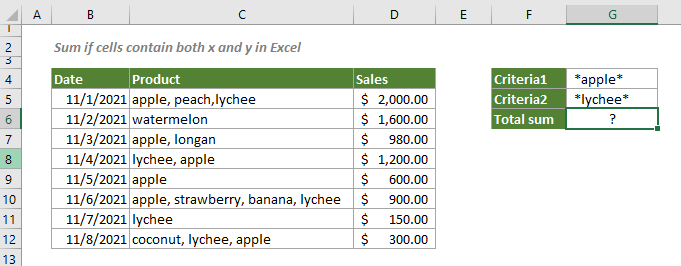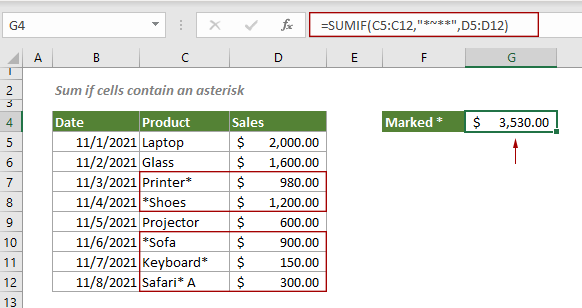## Sum if cells contain both x and y in Excel

This step-by-step tutorial demonstrates how to sum a range of cells if the corresponding cells containing both x and y by using the SUMIFS function.

#### How to sum if cells contain both x and y in Excel?

As shown in the screenshot below, if the cells in C5:C12 contain both “apple” and “lychee”, the corresponding cells in D5:D12 will be summed. You can follow the steps below to get it done.Generic Formulas

=SUMIFS(sum_range, criteria_range1, criteria1, criteria_range2, criteria2)

Arguments

Sum_range: The range of cells to sum;
Criteria_range1: The range of cells used to evaluate the criteria1;
Criteria_rang2: The range of cells used to evaluate the criteria2;
Criteria1: The criteria used to define which cells in Criteria_rang1 will be added.
Criteria2: The criteria used to define which cells in Criteria_rang2 will be added.

How to use this formula?

1. Select a blank cell, copy or enter the formula below and press the Enter key to get the result.

=SUMIFS(D5:D12, C5:C12, G4, C5:C12, G5)Notes:

1) In this formula, G4 and G5 are the cells containing the first and second criteria.
2) You can directly type a text value enclosed in double quotation marks as criteria like this:

=SUMIFS(D5:D12, C5:C12, “*apple*”, C5:C12, “*lychee*”)

3) As the SUMIFS function is based on AND logic by default, you can add more range and criteria pairs according to your needs like this:

SUMIFS(sum_range, criteria_range1, criteria1, [criteria_range2, criteria2], ...)

Explanation of the formula

=SUMIFS(D5:D12, C5:C12, G4, C5:C12, G5)

In this case, the SUMIFS function calculates numbers in column D. The first step is to specify the location of the numbers based on the given conditions.:
*apple*, *lychee*: Here we add asterisks at the start and end positions of the criteria to allow the formula to match “apple” and “lychee” no matter where they are in the cell.

#### Related functions

Excel SUMIFS function
The Excel SUMIF function can help to sum cell values based on multiple criteria.

#### Related formulas

Sum if cells contain an asterisk
To sum cells containing an asterisk that is regarded as a literal character not a wildcard, this tutorial explains a formula based on the SUMIF function and the tilde (~).

Sum if cells contain or equal to either x or y
To sum the cells if contain or equal to either one value or another, this tutorial provides two formulas in detail to help solve the problems.

Sum if date is between two dates
This tutorial demonstrates how to sum values within a certain date range in Excel with a formula based on the SUMIFS function.

Sum if cells are equal or not equal to a certain value
This step-by-step tutorial explains two formulas to sum a range of cells that equal or not euqal to a certain value based on the SUMIF function.

### The Best Office Productivity Tools

#### Kutools for Excel - Helps You To Stand Out From Crowd

 Popular Features: Find, Highlight or Identify Duplicates  |  Delete Blank Rows  |  Combine Columns or Cells without Losing Data  |  Round without Formula ... Super VLookup: Multiple Criteria  |  Multiple Value  |  Across Multi-Sheets  |  Fuzzy Lookup... Adv. Drop-down List: Easy Drop Down List  |  Dependent Drop Down List  |  Multi-select Drop Down List... Column Manager: Add a Specific Number of Columns  |  Move Columns  |  Toggle Visibility Status of Hidden Columns  |  Compare Columns to Select Same & Different Cells ... Featured Features: Grid Focus  |  Design View  |  Big Formula Bar  |  Workbook & Sheet Manager | Resource Library (Auto Text)  |  Date Picker  |  Combine Worksheets  |  Encrypt/Decrypt Cells  |  Send Emails by List  |  Super Filter  |  Special Filter (filter bold/italic/strikethrough...) ... Top 15 Toolsets:  12 Text Tools (Add Text, Remove Characters ...)  |  50+ Chart Types (Gantt Chart ...)  |  40+ Practical Formulas (Calculate age based on birthday ...)  |  19 Insertion Tools (Insert QR Code, Insert Picture from Path ...)  |  12 Conversion Tools (Numbers to Words, Currency Conversion ...)  |  7 Merge & Split Tools (Advanced Combine Rows, Split Excel Cells ...)  |  ... and more

Kutools for Excel Boasts Over 300 Features, Ensuring That What You Need is Just A Click Away...#### Office Tab - Enable Tabbed Reading and Editing in Microsoft Office (include Excel)

• One second to switch between dozens of open documents!
• Reduce hundreds of mouse clicks for you every day, say goodbye to mouse hand.
• Increases your productivity by 50% when viewing and editing multiple documents.
• Brings Efficient Tabs to Office (include Excel), Just Like Chrome, Edge and Firefox.